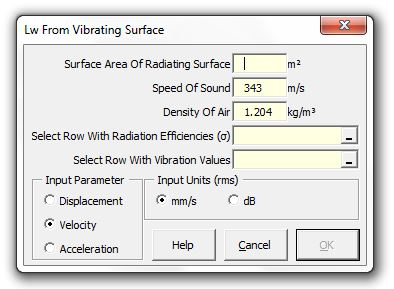### Strutt Help

Lw from Vibrating Surface   1/1, 1/3

Strutt|Vibration|Lw from Vibrating Surface calculates the sound power level of a vibrating surface.Strutt implements the formula as:

L_w = 10log((S rho_0 c_0 sigma v^2)/W_(ref))

where:
L_w is the radiated sound power level of the vibrating surface (dB re 1 pW)
v is the vibration velocity (m/s) of the vibration surface
S is the surface area (m²) of the vibrating surface
rho_0 is the density of air (kg/m³), by default 1.21 kg/m³
c_0 is the speed of sound in air (m/s), by default 343 m/s
sigma is the radiation efficiency of the vibrating surface (usually 1.0 above the critical frequency of the surface)
W_(ref) is the reference sound power level of 1*10^-12 (W)

Reference: Bies and Hansen Engineering Noise Control, Third Edition Section 6.7